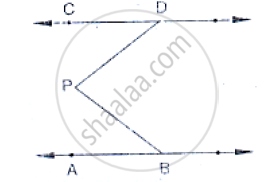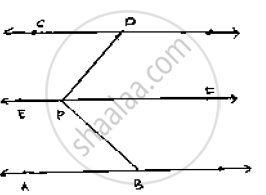# In the Below Fig, Lines Ab and Cd Are Parallel and P is Any Point as Shown in the Figure. Show that ∠Abp + ∠Cdp = ∠Dpb. - Mathematics

In the below fig, lines AB and CD are parallel and P is any point as shown in the figure.
Show that ∠ABP + ∠CDP = ∠DPB.#### SolutionGiven that AB || CD

Let EF be the parallel line to AB and CD which passes through P.

It can be seen from the figure

Alternative angles are equal

∠ABP = ∠BPF

Alternative angles are equal

∠CDP = ∠DPF

⇒ ∠ABP + ∠CDP = ∠BPF + ∠DPF

⇒ ∠ABP + ∠CDP = ∠DPB

Hence proved

AB parallel to CD, P is any point

To prove: ∠ABP + ∠BPD + ∠CDP = 360°

Construction : Draw  EF || AB passing through P

Proof:

Since AB || EF and  AB || CD

∴EF || CD       [Lines parallel to the same line are parallel to each other]

∠ABP + ∠EPB =180°      [Sum of co-interior angles is180° AB || EF and BP is the transversal]

∠EPD + ∠COP =180°

[Sum of co-interior angles is180° EF || CD and DP is transversal]                   …....(1)

∠EPD + ∠CDP =180°

[Sum of Co-interior angles is 180° EF || CD and DP is the transversal]                        …(2)

∠ABP + ∠EPB + ∠EPD + ∠CDP = 180° +180°

∠ABP + ∠EPB + ∠COP = 360°

Concept: Parallel Lines and a Transversal
Is there an error in this question or solution?

#### APPEARS IN

RD Sharma Mathematics for Class 9
Chapter 10 Lines and Angles
Exercise 10.4 | Q 24 | Page 49